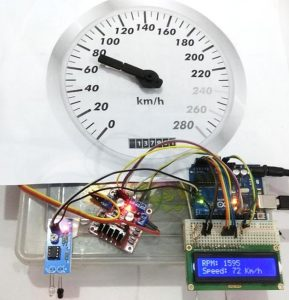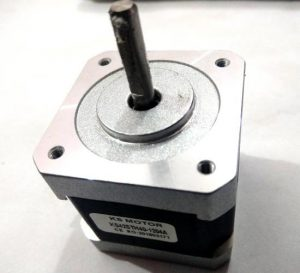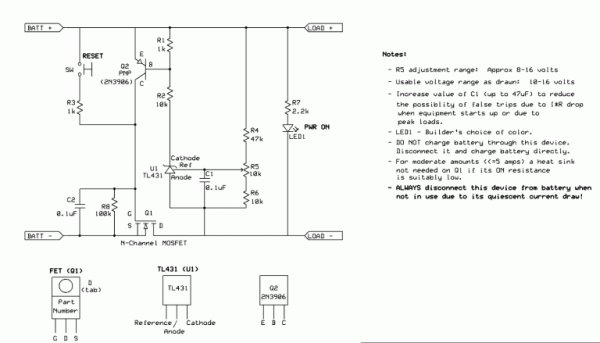# Analog Speedometer Using Arduino and IR SensorMeasuring the speed/rpm of a Vehicle or a motor has always been a fascinating project to try. In this project, we are going to build a Analog Speedometer using the Arduino. We will use IR Sensor module to measure the speed. There are other ways/sensors for this, like hall sensor to measure speed, but using an IR sensor is easy because IR sensor module is very common device and we can get it easily from the market and also it can be used on any type of motor/Vehicle.

In this project, we are going to show speed in both analog and digital form. By doing this project, we will also enhance our skills in learning Arduino and Stepper motor since this project involves use of Interrupts and Timers. At, the end of this project you will be able to calculate the speed and distances covered by any rotating object and display them on a 16×2 LCD screen in digital format and also on analog meter. So let’s start with this Speedometer and Odometer Circuit with Arduino

### Materials Required

1. Arduino
2. A bipolar stepper motor (4 wire)
3. Stepper motor driver (L298n Module)
4. IR sensor module
5. 16*2 LCD display
6. 2.2k resistor
7. Connecting wires
9. Power supply
10. Speedometer picture printout### Calculating Speed and Showing it on Analog Speedometer

An IR Sensor is a device which can detect the presence of an object in front of it. We have used two blade rotor (fan) and placed the IR sensor near it in such a way that every time the blades rotate the IR sensor detects it. We then use the help of timers and Interrupts in Arduino to calculate the time taken for one complete rotation of the motor.

READ  3D AIR mouse | Arduino + ProcessingIR Sensor

Here in this project, we have used highest priority interrupt to detect rpm and we have configured it in rising mode. So that whenever sensor output goes LOW to High, function RPMCount() will be executed. And as we have used two blade rotor, It means the function will be called 4 times in one revolution.

Once the time taken is known we can calculate the RPM by using the below formulae, Where 1000/time taken will give us the RPS (revolution per second) and further multiplying it with 60 will give you the RPM (revolution per minute)

After getting RPM, speed can be calculated by given formula:

Speed = rpm * (2 * Pi * radius) / 1000

We know that Pi = 3.14 and radius is 4.7 inch

But first we need to convert radius into meters from inches:

Speed= rpm * 60.0 * (2.0 * 3.14 * radius)/ 1000.0) in kilometers per hour

Here we have multiplied rpm by 60 to convert rpm to rph (revolution per hour) and divided by 1000 to convert meters/hour to Kilometers/hour.

After having speed in kmh we can show these values directly over the LCD in digital form but to show speed in the analog form we need to do one more calculation to find out no. of steps, stepper motor should move to show speed on analog meter.

Here we have used a 4 wire bipolar stepper motor for analog meter, which is having 1.8 degree means 200 steps per revolution.Now  we have to show 280 Kmh on speedometer. So to show 280 Kmh stepper motor needs to move 280 degree

So we have maxSpeed = 280

And maxSteps will be

maxSteps = 280/1.8 = 155 steps

Now we have a function in our Arduino code namely map function which is used here to map speed into steps.

Steps = map(speed,0,maxSpeed,0,maxSteps);

So now we have

steps=map(speed,0,280,0,155);

After calculating steps we can directly apply these steps in stepper motor function to move stepper motor. We also need to take care of current steps or angle of the stepper motor by using given calculations

currSteps=Steps
steps= currSteps-preSteps
preSteps=currSteps

here currSteps is current steps that is coming from last calculation and preSteps is last performed steps.

### Circuit Diagram and Connections

Circuit diagram for this Analog Speedometer is simple, here we have used 16×2 LCD to show speed in digital form and stepper motor to rotate the analog speedometer needle.

16×2 LCD is connected at following analog pins of Arduino.

RS – A5
RW – GND
EN – A4
D4 – A3
D5 – A2
D6 – A1
D7 – A0A 2.2k resistor is used to set the brightness of LCD. An IR sensor module, which is used to detect fan’s blade to calculate the rpm, is connected to interrupt 0 means D2 pin of Arduino.

Here we have used a stepper motor driver namely L293N module. IN1, IN2, IN3 and IN4 pin of stepper motor driver is directly connected to D8, D9, D10, and D11 of Arduino. Rest of connections are given in Circuit Diagram.### Programming Explanation

Complete code for Arduino Speedometer is given at the end, here we are explaining few important part of it.

In programming part, we have included all the required libraries like stepper motor library, LiquidCrystal LCD library and declared pins for them.

#include<LiquidCrystal.h>
LiquidCrystal lcd(A5,A4,A3,A2,A1,A0);
#include <Stepper.h>
const int stepsPerRevolution = 200; // change this to fit the number of steps per revolution
Stepper myStepper(stepsPerRevolution, 8, 9, 10, 11);

After this, we have taken some variables and macros for performing the calculations. Calculations are already explained in the previous section.

volatile byte REV;
unsigned long int rpm,RPM;
unsigned long st=0;
unsigned long time;
int ledPin = 13;
int led = 0,RPMlen , prevRPM;
int flag = 0;

int flag1=1;
int preSteps=0;
float stepAngle= 360.0/(float)stepsPerRevolution;
float minSpeed=0;
float maxSpeed=280.0;
float minSteps=0;
float maxSteps=maxSpeed/stepAngle;

After this, we initialize the LCD, Serial, interrupt and Stepper motor in the setup function

void setup()
{
myStepper.setSpeed(60);
Serial.begin(9600);
pinMode(ledPin, OUTPUT);
lcd.begin(16,2);
lcd.print(“Speedometer”);
delay(2000);
attachInterrupt(0, RPMCount, RISING);
}

After this, we read rpm in loop function and perform a calculation to get speed and convert that into steps to run stepper motor to show speed in analog form.

void loop()
{
int Speed= ((float)RPM * 60.0 * (2.0 * 3.14 * radius)/1000.0);
// RPM in 60 minute, diameter of tyre (2pi r) r is radius, 1000 to convert in km
int Steps=map(Speed, minSpeed,maxSpeed,minSteps,maxSteps);

if(flag1)
{
Serial.print(Speed);
Serial.println(“Kmh”);
lcd.setCursor(0,0);
lcd.print(“RPM: “);
lcd.print(RPM);
lcd.print(” “);
lcd.setCursor(0,1);
lcd.print(“Speed: “);
lcd.print(Speed);
lcd.print(” Km/h “);
flag1=0;
}
int currSteps=Steps;
int steps= currSteps-preSteps;
preSteps=currSteps;
myStepper.step(steps);
}

Here we have reapRPM() function to calculate RPM.

Analog Speedometer Using Arduino and IR Sensor

#### This Post / Project can also be found using search terms:

• speedometer using arduino
• which motor is used in speedometer
• analog speedometer using ardino and ir sensor circuit diagram
• analog speedometer using ardino and ir sensor programming code